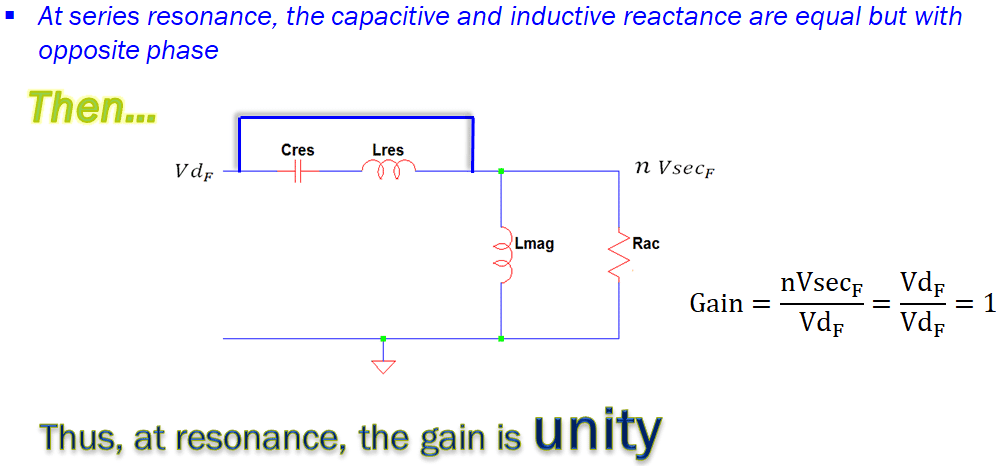# Why LLC Resonant Converter Gain is Unity

You may be wondering why LLC resonant converter gain is unity. How it happens and how to mathematically derive it. If you have these questions, then you are on the right page. I will be sharing to you the answers to these questions. In the previous topic, I discussed the equivalent AC circuit of an LLC resonant converter. Read How to Derive LLC Converter AC Circuit.

One of the main features of an LLC resonant converter is the ability to operate at resonance. At resonance, the power losses are low and the converter will attain soft switching. However, operation at resonance is needed to be designed through input and output voltage proper setting, selection of the right inductor and capacitor. When the LLC resonant converter operates outside resonance, its losses will be higher and its EMI performance will be affected.

## Here’s is the Explanation Why LLC Resonant Converter Gain is Unity

The general equation of a gain is output divided by input.

Gain = output / input

For the LLC equivalent circuit, the output side is the Rac section while the input side is the input voltage (Vdf) section. The voltage across Rac is turns ratio times the fundamental voltage across the transformer secondary winding.

Voltage at Rac = n x Vsecf

This is derived in the previous article LLC Resonant Converter AC Circuit Derivation.

Let us do the math. The input voltage, Vdf is given by below equation

Vin = Vdf = [4 x Vin / 2pi] [sin (2 x pi x f x t)]

On the other hand, the output voltage is can be elaborated as below

Vout = n x Vsecf = [n x 4 x Vo / pi] [sin (2 x pi x f x t)]

Gain = Vout / Vin = [n x 4 x Vo / pi] [sin (2 x pi x f x t)] / [4 x Vin / 2pi] [sin (2 x pi x f x t)] = 2 x n x Vo / Vin

Gain = 2 x n x Vo /Vin

The above gain equation is not unity but a function of turns ratio, output and input voltage. How the gain becomes unity then?

There is a twist…

At resonance, the capacitive and inductive reactance of Cres and Lres are equal but with opposite polarity. This will effectively results to a short circuit connection; literally replacing both the Cres and Lres with a wire. With this, the output and the input voltage is the same. Thus, the gain is unity.

During LLC tank circuit design, the gain equation, Gain = 2 x n x Vo / Vin is used to get the turns ratio by equating the gain to unity value.

For example, the Vo and Vin are 12V and 390V respectively, the turns ratio needed is

n = Gain x Vin  / ( 2 x Vo ) = (1) x 390V / ( 2 x 12V ) = 16.25

## Summary: Why LLC Resonant Converter Gain is Unity

1. LLC resonant converter ideal operation is at resonance. At resonance, the gain is unity.

2. The gain becomes unity because the inductive and capacitive reactance cancels each other at resonance.

3. The LLC resonant converter gain could be expressed as a function of turns ratio, output and input voltage.

Should you have a better explanation on why LLC resonant converter gain is unity, please share it here. Let’s discuss.

Please do not forget to hit the share and like button. Should you have any comments, suggestions or questions to improve this article, feel free to leave your comments. Let’s discuss! Electronics believer is also offering consultations, kindly email to [email protected].